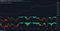The following oscillator uses an adaptive moving average as input for another RSI oscillator and aims to provide a way to minimize the impact of retracements over the oscillator output without introducing significant lag.

An additional trigger line is present in order to provide entry points from the crosses between the oscillator and the trigger line. More details are given below.

You can access the indicator here.

# Settings

• `Length `: period of the oscillator
• `Power `: control the sensitivity of the oscillator to retracements, with higher values minimizing the sensitivity to retracements.
• `Src `: source input of the indicator‌

The indicator also includes the following graphical settings:‌

• `Gradient` : determine the color mode to use for the gradient, options include "Red To Green", "Red To Blue" and "None", with "None" displaying no gradient.
• `Color fill` : determine whether to fill the area between the oscillator and the trigger line or not, by default "On".
• `Circles `: determine whether to show circles highlighting the crosses between the oscillator and the trigger line.

# Usage

The indicator can be used like any normalized oscillator, but unlike a classical RSI the oscillator does not converge toward 50 with higher length values, this is caused by the RSI using a smooth input.

The power setting will minimize the impact of certain variations on the oscillator:the oscillator at the bottom uses a power value of 1.5

The trigger line is a smoothed RSI using an EMA as input, and won’t remain as near to 100 and 0 as the main oscillator. Using a moving average of the main oscillator as a trigger line would create faster crosses, but’s this approach allows us to have no crosses when a retracement is present.

# Details

As previously discussed the main oscillator uses an adaptive moving average as input; this adaptive moving average is computed using a smoothing factor derived from an RSI oscillator, a similar adaptive moving average known as ARSI, but unlike ARSI which uses a classical RSI of the closing price for the calculation of the smoothing factor, our smoothing factor makes use of RSI on the adaptive moving average error, which provides a higher level of adaptiveness.

Written by